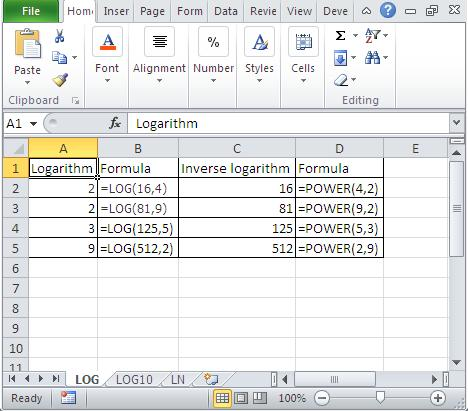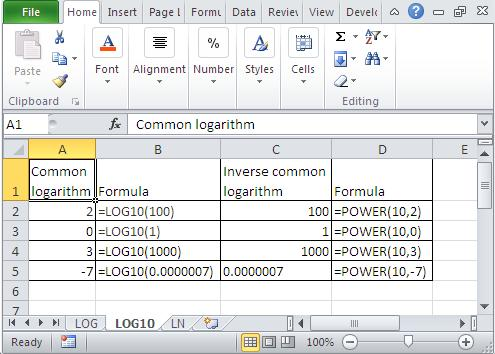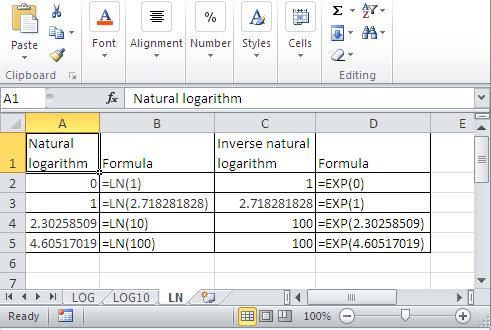#### How to calculate logarithms and inverse logarithms in Excel

Logarithms and inverse logarithms are mathematical functions that are used in many different fields, including mathematics, engineering, and finance. We will teach you how to calculate logarithms and inverse logarithms in Excel.

We will start by explaining what logarithms are and how they work. Then, we will show you how to use the LOG, LOG10, and LN functions in Excel to calculate logarithms and inverse logarithms. Finally, we will provide you with some examples of how to use these functions in real-world applications.

By the end of this article, you will be able to calculate logarithms and inverse logarithms in Excel with ease.

## What is a logarithm?

Logarithms are mathematical functions that help us to understand the relationship between two numbers. The easiest way to understand logarithms is to understand this mathematical formula:

Loga b=c ac=b

For example

Log2 3 = 8 because 23=8

In this example, the base is 2, the exponent is 3, and the value is 8. This means that 2 raised to the power of 3 is equal to 8.

## What is a common logarithm?

A common logarithm is a logarithm with a base of 10. This means that log10 x is the power to which 10 must be raised to equal x. You don’t need to write 10 as a base. Log10 x = log x

For example:

log 1 = 0

log 10 = 1

log 1000 = 3

## What is a natural logarithm?

A natural logarithm is a logarithm with a base of e, which is a mathematical constant approximately equal to 2.718281828459. This means that ln x is the power to which e must be raised to equal x. For example, ln 100 = 4.605170185988092 because e raised to the power of 4.605170185988092 is equal to 100.

Microsoft Excel has built-in functions to calculate the logarithm of a number with a specified base, the logarithm with base 10, and the natural logarithm. To calculate the inverse log of a number in the first two cases, raise the base to the power of the value returned by the particular logarithm function being used. Use the EXP function to find the inverse natural log.

## How to calculate a logarithm and inverse of a logarithm?

To calculate the logarithm of a number in Excel, use the LOG function.

The syntax is =LOG(n,b), where n is the number and b is the base.

For example, =LOG(16,4) will return the value 2 because 4 raised to the power of 2 is equal to 16.

=LOG(16,4) – result is 2

=LOG(81,9) – result is 2

=LOG(125,5) = result is 3

=LOG(512,2) – result is 9

To calculate the inverse logarithm of a number, use the POWER function.

The syntax is =POWER(b,result), where b is the base and result is the value returned by the LOG function.

For example, =POWER(4,2) will return the value 16 because 4 raised to the power of 2 is equal to 16.

=POWER(4, 2) – equals 16

=POWER(9, 2) – equals 81

=POWER(5, 3) – equals 125

=POWER(2, 9) – equals 512## How to calculate a common logarithm and inverse of common logarithm?

To calculate the common logarithm of a number in Excel, use the LOG10 function.

The syntax is =LOG10(n), where n is the number.

For example, =LOG10(100) will return the value 2 because 10 raised to the power of 2 is equal to 100.

=LOG10(100) – result is 2

=LOG10(1) – result is 0

=LOG10(1000) = result is 3

=LOG10(0.0000001) – result is -7

To calculate the inverse of a common logarithm, use the POWER function.

The syntax is =POWER(10,result), where result is the value returned by the LOG10 function.

For example, =POWER(10,2) will return the value 100 because 10 raised to the power of 2 is equal to 100.

=POWER(10, 2) – equals 100

=POWER(10, 0) – equals 1

=POWER(10, 3) – equals 10

=POWER(10, -7) – equals 0.0000001## How to calculate a natural logarithm and inverse of natural logarithm?

To calculate the natural logarithm of a number in Excel, use the LN function.

The syntax is =LN(n), where n is the number.

For example, =LN(10) will return the value 2.30258509 because e raised to the power of 2.30258509 is equal to 10.

=LN(1) – result is 0

=LN(2.718281828) – result is 1

=LN(10) = result is 2.30258509

=LN(100) – result is 4.60517019

To calculate the inverse of a natural logarithm, use the EXP function.

The syntax is =EXP(n), where n is the value returned by the LN function.

For example, =EXP(2.30258509) will return the value 10 because e raised to the power of 2.30258509 is equal to 10.

=EXP(0) – equals 1

=EXP(1) – equals 2.718281828

=EXP(2.30258509) – equals 10

=EXP(4.60517019) – equals 100## How to get rid of ln?

To undo LN in Excel is to use EXP, which reverses the natural logarithm function.

For example:

If you have a cell with =LN(10) and want to undo LN, do this:

1. Click on the cell with the formula.
2. Type = and then EXP( to start the exp function.
3. Select the cell with the LN function (the one with =LN(10)).
4. Type ) to end the exp function.

Your new formula should be =EXP(A1) (if the cell with LN is A1).

Press enter and the formula will give the result of EXP, which is the inverse of LN. In this case, the result would be 22026.47, which is e^ln(10).